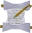## FANDOM

44,133 PagesTemplate documentation [purge]
This documentation is transcluded from Template:SimpleGoal/doc.

The SimpleGoal template displays a completion bar based on the amount of the goal that has currently been achieved. For skill goals, see Template:Goal.

## Usage

```{{SimpleGoal
|Label     = optional label (replaces image of coins)
|Goal      = target number
|Have      = current number
|Units     = units to display after the amount to go (e.g "gp")
|Width     = overall width (default 300 pixels)
|BarColor1 = color of achieved part of progress bar (default gold)
|BarColor2 = color of remaining part of progress bar (default dark red)
|BarWidth  = width of bar (default 110 pixels)
}}
```

## Examples

Below are some examples of the various uses of the SimpleGoal template. The first example gives the desired number of coins and the number so far:

`{{SimpleGoal | Goal=1000000 | Have=215000 | Units=gp }}`

The example produces the following result:

The second example gives the desired number of music songs and the number so far:

```{{SimpleGoal | Label=[[File:Music icon.png]] | BarColor1=darkcyan
| Goal=500 | Have=312 | Units=songs }}```

The example produces the following result:

The third example gives the desired number of quest points and the number so far:

```{{SimpleGoal | Label=[[File:Quest.png]] | BarColor1=green | BarColor2=blue
| Goal=270 | Have=256 | Units=qp }}```

The example produces the following result:

The fourth example gives the quest points, but with the widths specified to match the size of the Goal template:

```{{SimpleGoal | Label=[[File:Quest.png]] | BarColor1=green | BarColor2=blue
| Goal=270 | Have=256 | Units=qp | Width=441 | BarWidth=201 }}```

The example produces the following result:

Community content is available under CC-BY-SA unless otherwise noted.